﻿ 汽车天窗耦合风振噪声规律及机理研究 Research on Regularity and Mechanism of Vehicle Coupling Sunroof Buffeting Noise

Mechanical Engineering and Technology
Vol. 08  No. 02 ( 2019 ), Article ID: 29579 , 10 pages
10.12677/MET.2019.82009

Research on Regularity and Mechanism of Vehicle Coupling Sunroof Buffeting Noise

Daren Zhou1,2, Yuming Peng1,2

1Engineering Research Center of Advanced Driving Energy-Saving Technology, Ministry of Education, Chengdu Sichuan

2Southwest Jiaotong University, Chengdu SichuanReceived: Mar. 8th, 2019; accepted: Mar. 27th, 2019; published: Apr. 3rd, 2019ABSTRACT

Wind buffeting noise as popular problem exists in the vehicle driving process when windows opened. In this paper, the characteristics of sunroof buffeting noise are studied by LBM-LES simulation. The sunroof buffeting noise under conditions of different speeds, different opening size and different combined opening pattern of sunroof and side-window is simulated and analyzed. The results demonstrate that the most obvious phenomenon of sunroof buffeting occurs at the speed which Helmholtz resonance arises. The buffeting noise increases with the increase of sunroof opening size. The mechanism of sunroof buffeting is that the periodic pressure fluctuation of the cabin is caused by periodic vortex shedding in the shear layer of sunroof opening. The “aeration effect” does not exist when the left-front side window and sunroof are opened. Because of the direct action of fluid, in the direction perpendicular to the open window, the buffeting noise increases from the window to the inside.

Keywords:Vehicle, LBM-LES, Sunroof, Buffeting Noise, Coupling

1先进驱动节能技术教育部工程研究中心，四川 成都

2西南交通大学，四川 成都1. 引言

2. 数值计算方法

2.1. 格子玻尔兹曼方法

$\frac{\partial {f}_{i}}{\partial t}+{c}_{i}\cdot \nabla {f}_{i}+\frac{F}{m}\cdot \frac{\partial {f}_{i}}{\partial {c}_{i}}={\Omega }_{i}\left(f\right)$ (1)

${\Omega }_{i}\left(f\right)=\omega \left({f}_{i}{}^{eq}-{f}_{i}\right)=\frac{1}{\tau }\left({f}_{i}{}^{eq}-{f}_{i}\right)$ (2)

2.2. 大涡模拟

LBM方法数值耗散低，LES采用亚格子模型。本文使用的WALE模型，涡粘度定义如下：

${v}_{t}=\rho {L}_{s}^{2}\frac{{\left({S}_{ij}^{d}{S}_{ij}^{d}\right)}^{\frac{3}{2}}}{{\left({\overline{S}}_{ij}{\overline{S}}_{ij}\right)}^{\frac{5}{2}}+{\left({S}_{ij}^{d}{S}_{ij}^{d}\right)}^{\frac{5}{4}}}$ (3)

${S}_{ij}^{d}=\frac{1}{2}\left({\overline{g}}_{ij}^{2}+{\overline{g}}_{ji}^{2}\right)-\frac{1}{3}{\delta }_{ij}{\overline{g}}_{kk}^{2}$ (4)

${\overline{g}}_{ij}=\frac{\partial {v}_{i}}{\partial {x}_{j}}$ (5)

3. 数值计算方案

3.1. 计算域及格子尺度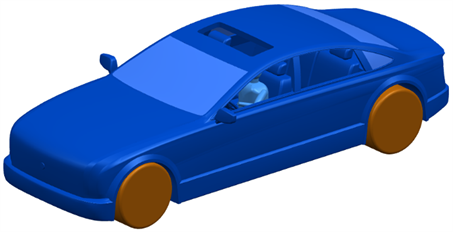Figure 1. Vehicle modelFigure 2. Computational domain model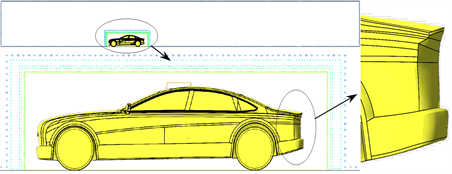Figure 3. Initial particle distribution in computational domain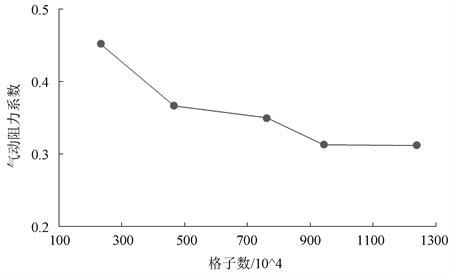Figure 4. Particle independence verification

3.2. 计算方法

4. 天窗风振噪声分析

4.1. 不同来流速度下天窗风振噪声分析

${f}_{a}=\frac{c}{2\pi }\sqrt{\frac{S}{V\left(h+\frac{\pi {D}_{h}}{4}\right)}}$ (6)

${f}_{b}=\frac{1}{3}\left(n-\frac{1}{4}\right)\frac{u}{{L}_{N}}$ (7)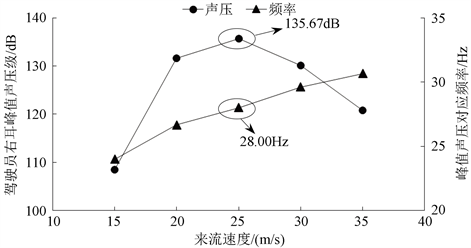Figure 5. Variation of peak sound pressure and frequency with different velocityFigure 6. Sound pressure spectrogram of each monitoring point at 25 m/s

4.2. 不同开度下天窗风振噪声分析

4.3. 天窗风振噪声机理分析

$P=\frac{F}{l}=\rho \Gamma \left(t\right){u}_{c}/l$ (8)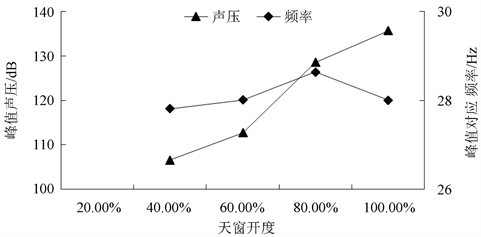Figure 7. Variation of peak sound pressure and frequency with different openings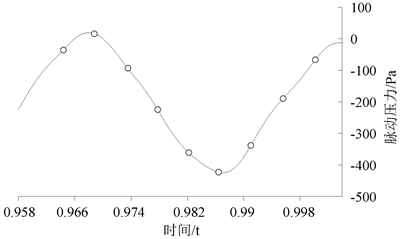Figure 8. Fluctuation pressure in driver’s right ear in a period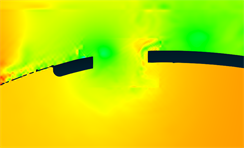(a) t = 0.9645 s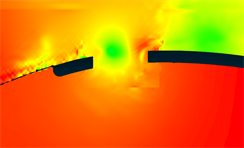(b) t = 0.9689 s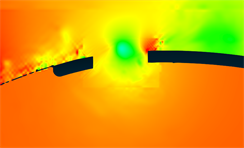(c) t = 0.9737 s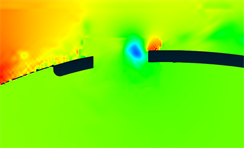(d) t = 0.9778 s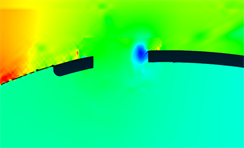(e) t = 0.9825 s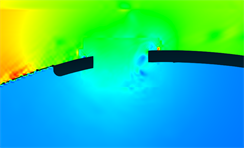(f) t = 0.9869 s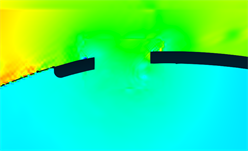(g) t = 0.9914 s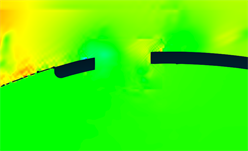(h) t = 0.9958 s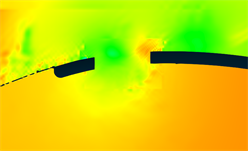(i) t = 1.0002 s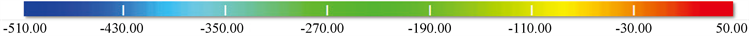Figure 9. Static pressure distribution clouds of passenger chamber

4.4. 天窗、侧窗耦合风振噪声分析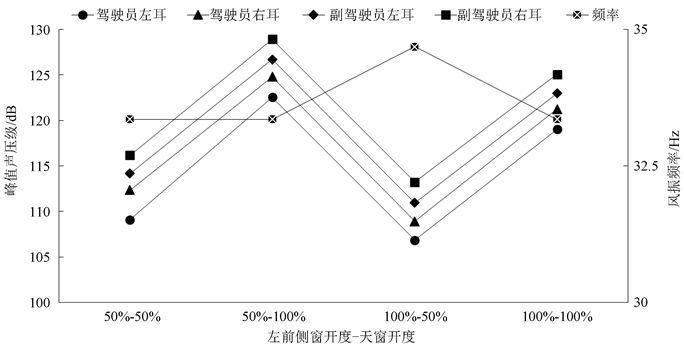Figure 10. Variation of peak sound pressure and frequency with different combined opening pattern of left-front side window and sunroofFigure 11. Variation of peak sound pressure and frequency with different combined opening pattern of left-rear side window and sunroof

5. 结论

1) 汽车天窗风振噪声随来流速度的增加先增强后减弱，对于本款车当来流速度为25 m/s时，剪切层的脱涡频率与乘员室腔体固有频率相接近，发生Helmholtz共振，产生风振噪声最强，峰值声压为135.67 dB，对应频率为28.00 Hz；

2) 天窗风振噪声随天窗开度的增大而增大。对于本款车当来流速度为25 m/s时，当天窗开度小于40%时几乎无明显风振现象，当天窗开度大于60%时，风振现象明显；

3) 天窗风振噪声产生机理：剪切层涡的周期性脱落及其后续的发展过程致使乘员室腔体内压力脉动呈周期性变化；

4) 监测点被流体直接作用时，产生的噪声与风振噪声相叠加，风振现象较弱，垂直于车窗开口向内声压峰值依次递增，流体不直接作用监测点时，声压峰值几乎相等；

5) “通风效应”只出现在车窗“非平衡”开启的工况，车窗“平衡”开启工况与车窗单开时声压峰值变化幅度不大。

Research on Regularity and Mechanism of Vehicle Coupling Sunroof Buffeting Noise[J]. 机械工程与技术, 2019, 08(02): 61-70. https://doi.org/10.12677/MET.2019.82009

1. 1. 谷正气, 肖朕毅, 莫志姣. 汽车风振噪声的CFD仿真研究现状[J]. 噪声与振动控制, 2007, 27(4): 65-68.

2. 2. An, C.F. and Singh, K. (2007) Sunroof Buffeting Suppressing Using a Dividing Bar. SAE Paper 2007-01-1552.

3. 3. Bodger, W.K. and Jones, C.M. (1964) Aerodynamic Wind Throbin Passenger Cars. SAE Technical Paper 640797.

4. 4. An, C.F., Alaie, S.M. and Sovani, S.D. (2004) Side Window Buffeting Characteristics of an SUV. SAE Paper 2004-01-0230. https://doi.org/10.4271/2004-01-0230

5. 5. An, C.F. and Singh, K. (2006) Optimization Study for Sunroof Buffeting Reduction. 2006 SAE Word Congress, Warrendale, 3-6 April 2006, 1-8. https://doi.org/10.4271/2006-01-0138

6. 6. 康宁, 王晓春. 天窗对轿车内部流场及气动噪声的影响[J]. 航空动力学报, 2010, 25(2): 354-358.

7. 7. 胡亚涛, 薛永飞, 陈军, 等. 车辆天窗气动噪声的数值分析与实验研究[J]. 工程热物理学报, 2011, 32(4): 589-592.

8. 8. Lekkerkerker, H.N.W., Poon, C.K., Pusey, P.N., et al. (1996) Lattice BGK Models for Navier-Stokes Equation. Europhysics Letters, No. 6, 479-484.

9. 9. 杨振东, 谷正气. 基于尺度自适应模拟的汽车天窗风振噪声特性分析[J]. 机械工程学报, 2016, 52(12): 107-117.

10. 10. Blevins, R.D. (1990) Flow-Induced Vibration. Van Nostrand Reinhold, New York.

11. 11. Kook, H.S. and Mongeau, L. (2002) Analysis of the Periodic Pressure Fluc-tua-tions Induced by Flow over a Cavtiy. Journal of Sound and Vibration, 251, 823-846. https://doi.org/10.1006/jsvi.2001.4013